# Chemistry formula for class 12 chapter- Liquid Solutions

#### Chemistry Formulas

The solution combines solute and solvent. It is defined as a homogeneous mixture of two or more substances. They can be divided into three types:

1. Solid solutions
2. Gaseous solutions
3. Liquid solutions

## Henry's Law

In liquids, gas separation is circulated by Henry's law. It states that the dissolution of gas in a liquid at a given temperature is directly related to the partial pressure of the gas. Due to the presence of a constant solute, the vapor pressure of the solvent decreases.

### Raoult's Law

Raoult's law circulates the reduction of vapor solvent. The law states that the corresponding reduction in the vapor pressure of a soluble substance over the solution and the fraction of the fixed solute molecule present in the solution is equal. It is expressed as follows:

ptotal = p10x1 + p20x2

• Solution: A homogeneous mixture of two or more substances is known as solution.
• Solute: The substance present in smaller amount in a solution is called solute.
• Solvent: The substance present in larger amount in a solution is called solvent.
• Binary solution: Theose solutions which contain two components are called binary solutions, e.g., salt solution, benzene and toluene.
• Ternary solutions: Those solutions which contain three components are called ternary solutions, e.g., ethanol + water + acetic acid.
• Aqueous solution: When solute is dissolved in water, it is called aqueous solution, e.g., sugar solution, ethanol is water.
• Non-aqueous solution: when solute is dissolved in solvent other than water, it is called non-aqueous solution, e.g., iodine dissolved in alcohol (Tincture of iodine).
• Weight fraction =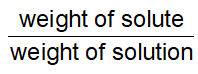• Weight percentage=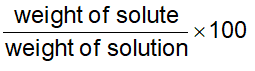Weight of solute present in 100 grams of solution is called weight percentage

Volume of solute present in 100 ml of the solution is called volume percentage

• Volume percentage =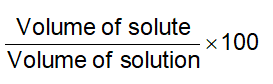• Volume Fraction =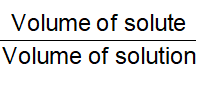• Molarity (M) =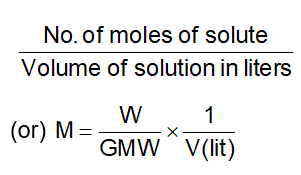• Molarity=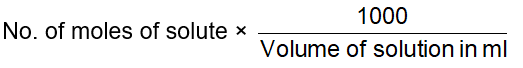• Molarity=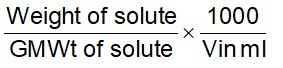• Weight of solute =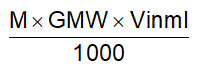In chemistry, the concentration of the solution is how much of the dissoluble substance, known as solute, is mixed with another substance, called a solvent. The standard formula is C = m / V, where C is the concentration, m is the mass of the solute, and V is the total volume of the solution.

### Ideal Solutions

The solutions which obey Raoult’s law are called ideal solutions. When the forces of attraction between A – A, B – B is similar to A – B, then A and B will from ideal solution. There are three specific properties of ideal solution :

1. Raoult’s law is obeyed i.e., PTOTAL = PA - PB
2. Hmix = 0
3. Vmix = 0

E.g. ; n–hexane and n–heptane; ethyl bromide and ethyl iodide; benzene and toluene; chlorobenzene and brompbenzene form ideal solutions.

### Non–ideal Solutions

Those solutions which do not obey Raoult’s law. When the force of attraction between A – A, B – B is different from A – B then ‘A’ and ‘B’ from non–ideal solutions. For these solutions :

1. Raoult’s law is not obeyed
2. Hmix is not equal to 0
3. Vmix is not equal to 0.

E.g., ethanol and water, chloroform and acetone, ethanol and cyclohexane are few examples of non–ideal solutions.

#### Positive Deviation from Raoult’s law

In those non–ideal solutions, in which partial pressure of each component A and B is higher than that calculated from Raoult’s law show positive deviation from Raoult’s law, as shown in figure e.g., water and ethanol, chloroform and water water, ethanol and CCl4, methanol and chloroform, benzene and methanol, acetic acid and toluene, acetone and ethanol, methanol and H2O

#### Negative deviation from Raoult’s Law

The partial vapour pressure of component ‘A’ is found to be less than caculated from Raoult’s law on adding the second compound ‘B’ and when A is added to B, the partial vapour pressure of component ‘B’ is found to be less than that calculated from Raoult’s law. The total vapour pressure of solution is less than that of ideal solution of same composition. Boiling point of such a solution is relative higher than the boiling points of A and respectively.

This type of deviation from ideal behaviour is known as negative deviation from Raoult’s law, as shown in figure. e.g., chloroform and acetone, chlororform and methyl acetate, H2O and HCl, H2O and HNO3, acetic acid and pyridine, chlororoform and benzene.

#### Azeotropes

Liquid mixture which distill over without changes in composition are called constant boiling mixture or Azeotropes or Axeotropic mixture.

#### Minimum Boiling Azeotropes

Non-ideal solutions showing large positive deviation from Raoult’s law from minimum boiling azeotropes which boil at temperature lower than boiling point of its components ‘A’ and ‘B’, e.g., water and benzene, chloroform and methanol.

#### Maximum Boiling Azeotropes

Non-ideal solutions showing large negative deviation from Raoult’s law from maximum boiling azeotropes which boil at temperature higher than the boiling point of its components A and B respectively, e.g., a mixture of HCl and H2O containing 20.2% HCl by weight boils at 108.5°C higher than either pure HCl (–85°C) or eater (100°C).

Do find the detail NCERT Solutions for Class 12 Chemistry and Class 12 Chemistry Notes prepared by expert faculty of Physics Wallah.

### Also Check

Download free pdf sheet which consist of s and important points of Liquid Solutions given below.